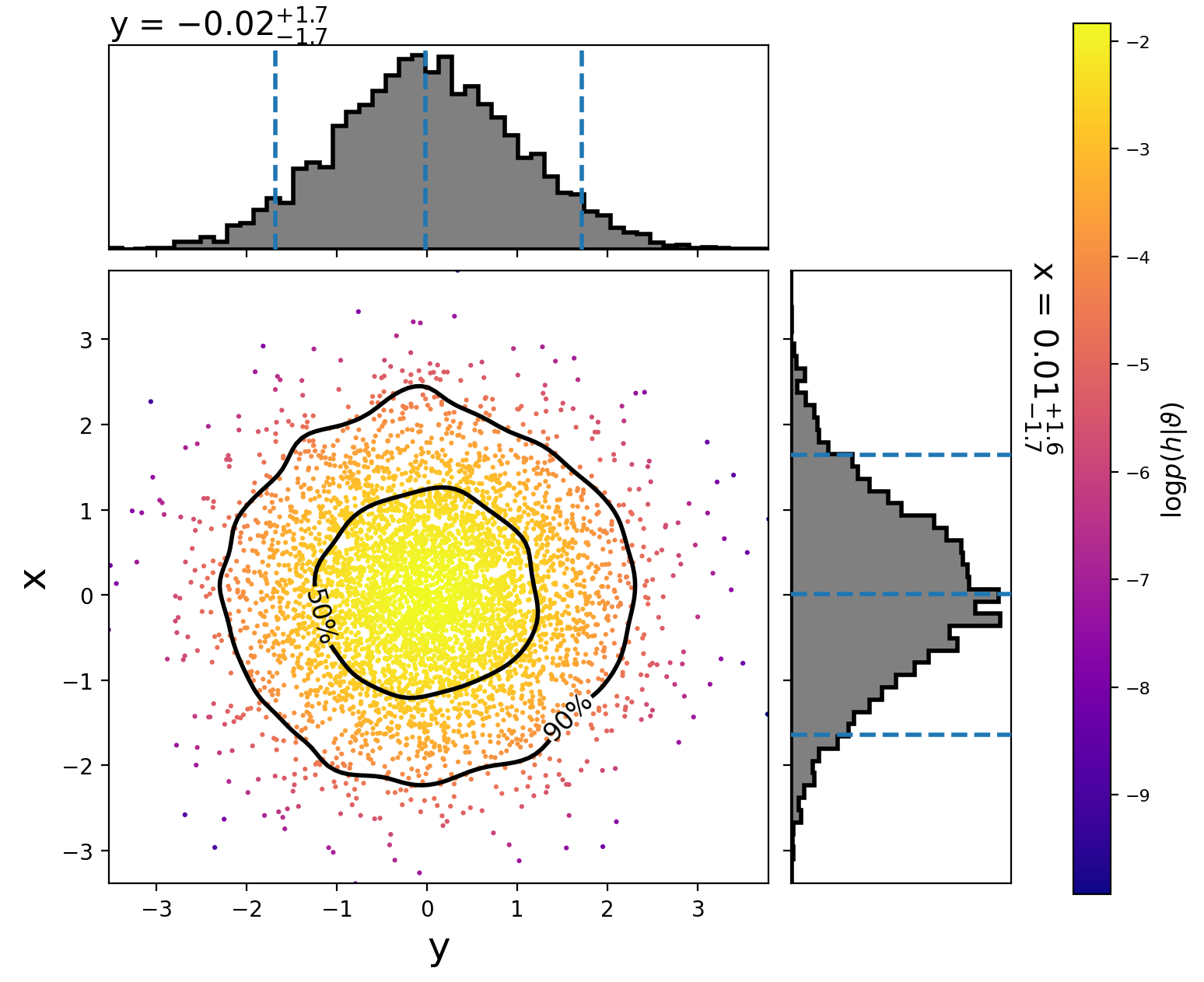# Running on an analytic distribution¶

Several analytic distributions are available to run tests on. These can be run quickly on a laptop to check that a sampler is working properly.

This example demonstrates how to sample a 2D normal distribution with the `emcee` sampler. First, we create the following configuration file:

```[model]
name = test_normal

[sampler]
name = emcee
nwalkers = 5000
niterations = 100

[variable_params]
x =
y =

[prior-x]
name = uniform
min-x = -10
max-x = 10

[prior-y]
name = uniform
min-y = -10
max-y = 10
```

`Download`

By setting the model name to `test_normal` we are using `TestNormal`. The number of dimensions of the distribution is set by the number of `variable_params`. The names of the parameters do not matter, just that just that the prior sections use the same names.

Now run:

```#!/bin/sh
pycbc_inference --verbose \
--config-files normal2d.ini \
--output-file normal2d.hdf \
--nprocesses 2 \
--seed 10 \
--force
```

`Download`

This will run the `emcee` sampler on the 2D analytic normal distribution with 5000 walkers for 100 iterations. When it is done, you will have a file called `normal2d.hdf` which contains the results. It should take about a minute to run. If you have a computer with more cores, you can increase the parallelization by changing the `nprocesses` argument.

To plot the posterior distribution after the last iteration, run:

```#!/bin/sh
pycbc_inference_plot_posterior --verbose \
--input-file normal2d.hdf \
--output-file posterior-normal2d.png \
--plot-scatter \
--plot-contours \
--plot-marginal \
--z-arg 'loglikelihood:\$\log p(h|\vartheta)\$' \
--iteration -1
```

`Download`

This will create the following plot:The scatter points show each walker’s position after the last iteration. The points are colored by the log likelihood at that point, with the 50th and 90th percentile contours drawn.

See below for more information about using `pycbc_inference_plot_posterior`.

To make a movie showing how the walkers evolved, run:

```#!/bin/sh
pycbc_inference_plot_movie --verbose \
--nprocesses 4 \
--input-file normal2d.hdf \
--output-prefix frames-normal2d \
--movie-file normal2d_mcmc_evolution.mp4 \
--cleanup \
--plot-scatter \
--plot-contours \
--plot-marginal \
--z-arg 'loglikelihood:\$\log p(h|\vartheta)\$' \
--frame-step 1
```

`Download`

Note

You need `ffmpeg` installed for the mp4 to be created.